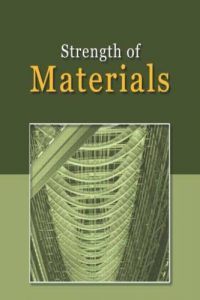اسم المؤلف
التاريخ
15 يناير 2020
المشاهدات
24
التقييم(لا توجد تقييمات)Loading...

Strength of Materials
Second Class Lecturer
College of Engineering
Mech. Eng. Dept.
Contents
Simple Stress
Tension Compression
Normal stress
Normal Strain
Double shear stress
Young’s modulus.
Poisson’s Ratio
Longitudinal Strain
Lateral Strain
Modulus of Rigidity ( G )
Statically Indeterminate Members
Thermal Stresses
T: Change in temperature of the member.
L: The original length of the member.
T: The change in length of the member
Initial Position
Final Position
Steel Aluminum Steel
Torsion
The Torsion Formula
T: The resultant internal torque acting on the cross section.
J: The polar moment of inertia of the cross sectional area.
Angle of Twist
G: The shear modulus of elasticity or modulus of rigidity
Torsion of Solid Noncircular Shafts
Shape of cross section
Thin walled tubes having closed cross sections
Average shear stress
The average shear stress acting over the thickness of the tube.
T: The resultant internal torque at the cross section.
t: The thickness of the tube where
avg is to be determined.
Am: The mean area enclosed within the boundary of the center line of the tube thickness
Angle of Twist
Thin Walled Cylinder, Thin Walled Pressure Vessels
a. Circumferential or hoop stress
b. Longitudinal or axial stress
Circumferential or hoop strain
d. Longitudinal strain
longitudinal strain
Change in internal volum
longitudinal strain
diametral strain
2. Spherical Vessels
Cylindrical Vessels with Hemispherical Ends
a) For the cylindrical portion
hoop stress
longitudinal stress
hoop strain
b) For the spherical ends
hoop stress
hoop strain
Shear and Moment Diagram
Concentrated force
Sign Convention
Procedure of Analysis
Location of maximum moment
Graphical Method for Constructing Shear and Moment Diagram
Stresses in Beams
S.F. Diagram
B.M. Diagram
Nonuniform bending Pure bending
Assumptions:
1. The beam is initially straight and unstressed.
2. The material of the beam is perfectly homogeneous.
3. The elastic limit is nowhere exceeded.
4. Young’s modulus for the material is the same in tension and compression.
5. Plane cross-sections remain plane before and after bending.
6. Every cross-section of the beam is symmetrical about the plane of bending i.e. about
an axis perpendicular to the N.A.
Composite Beams
Transformation factor (modular ratio)
Normal stress at the bar’s top.
Normal stress at the bar’s bottom
Slop and Deflection in Beams
Sign convention and coordinates
Boundary conditions
Castigliano’s Theorem Applied to Beams
Statically Indeterminate Beams
1. Method of Integration
Moment Area Method(Statically Indeterminate)
Combined Stresses
1. Axial and flexural.
2. Axial and torsional.
3. Torsional and flexural.
4. Axial , torsional and flexural.
Stresses at a Point
General State of Stress Plane Stress
Mohr’s Circle
Principal Stresses
Maximum in Plane Shear Stress
Stress on Arbitrary Plane
Stresses Due to Axial Load and Torsion
Mohr’s circle or from stress transformation equations.
Theories of Failure
Ductile Materials
Maximum Shear Stress Theory
Guest-Tresca
Maximum Principal Strain Theory
Saint-Venant
Maximum Shear Strain Energy Per Unit Volume (Distortion Energy Theory)
Triaxial stress
(Maxwell-Huber-Von Mises)
Total Strain Energy Per Unit Volume
Maximum shear stress theory
Columns
كلمة سر فك الضغط : books-world.net